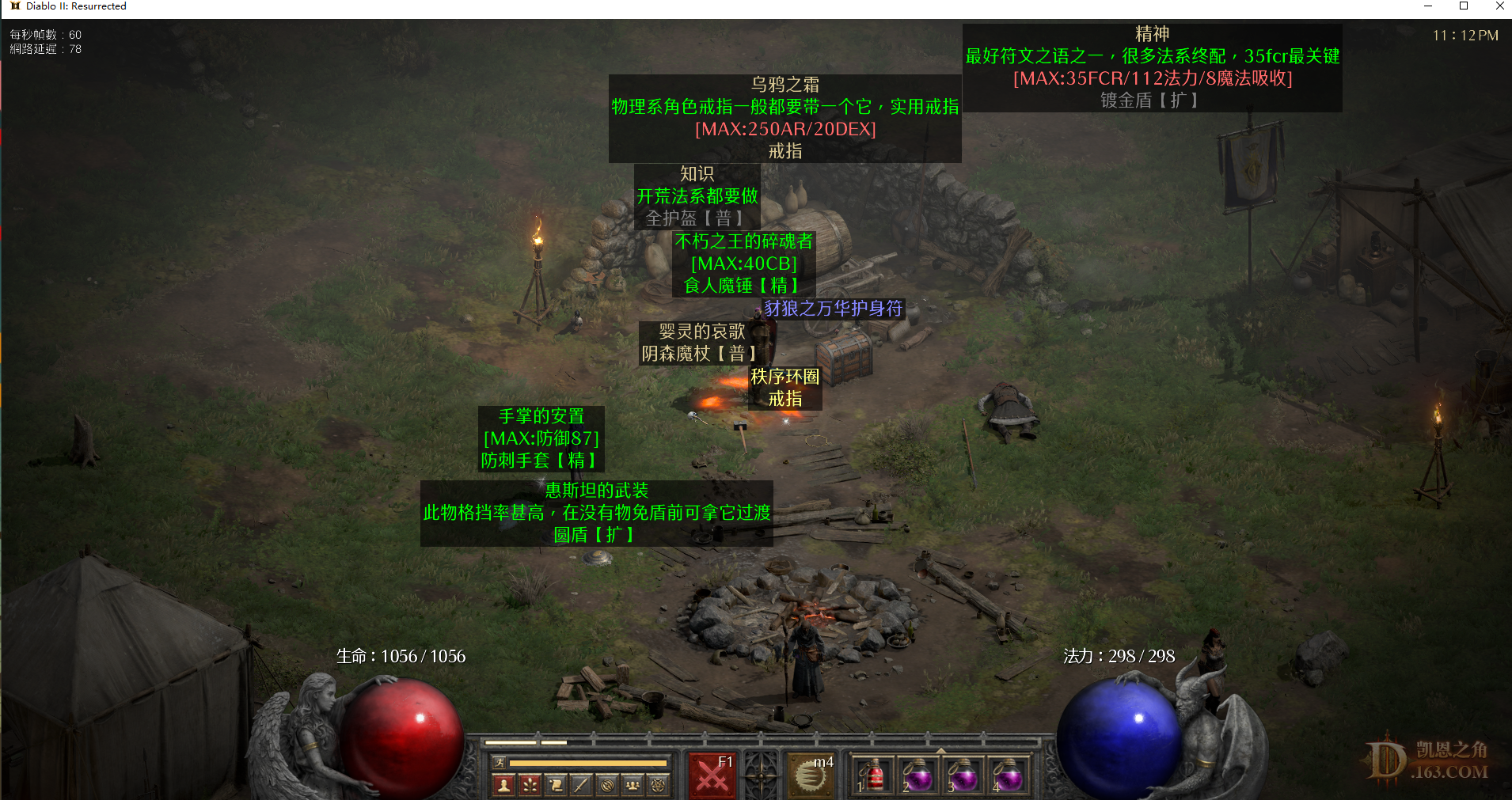//没登陆

 本帖最后由 河西因扎吉 于 2021-11-14 23:32 编辑 已送完#24=5     #25=7     #26=6     #27=2     #28=6
#29=1     #30=1     #31=0     #32=0     #33=0

 谢谢，我拿了一个乌鸦，刚好很需要~

 我是小透明 发表于 2021-11-14 23:18 谢谢，我拿了一个乌鸦，刚好很需要~ 能用到就好，今天打出来两个乌鸦

#24=5     #25=7     #26=6     #27=2     #28=6
#29=1     #30=1     #31=0     #32=0     #33=0

 进不去呢 ~~~~~~~~~~~~~~~

Nice#22138
 河西因扎吉 发表于 2021-11-14 23:19 能用到就好，今天打出来两个乌鸦 都哪里掉的啊~

 我是小透明 发表于 2021-11-14 23:23 都哪里掉的啊~ 现在在K3C

#24=5     #25=7     #26=6     #27=2     #28=6
#29=1     #30=1     #31=0     #32=0     #33=0

 东西还有吗，需要个精神

Nice#22138
 奈斯吖 发表于 2021-11-14 23:27 东西还有吗，需要个精神 不好意思哈，已经送完了

#24=5     #25=7     #26=6     #27=2     #28=6
#29=1     #30=1     #31=0     #32=0     #33=0

 河西因扎吉 发表于 2021-11-14 23:32 不好意思哈，已经送完了 好的，Nice#22138